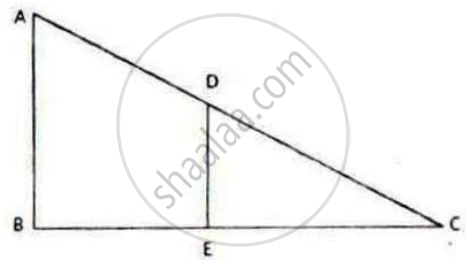Share

# In the Given Figure, Ab and De Are Perpendicular to Bc. 1) Prove that δAbc ∼ δDec 2) If Ab = 6 Cm; De = 4 Cm and Ac = 15 Cm. Calculate Cd. 3) Find the Ratio of Area of δAbc: Area of δDec - ICSE Class 10 - Mathematics

#### Question

In the given figure, AB and DE are perpendicular to BC.1) Prove that ΔABC ∼ ΔDEC

2) If AB = 6 cm; DE = 4 cm and AC = 15 cm. Calculate CD.

3) Find the ratio of area of ΔABC: area of ΔDEC

#### Solution

1) In ΔABC and ΔDEC,

∠ABC = ∠DEC = 90°

∠ACB = ∠DCE (Common)

∴ ΔABC ~ ΔDCE (AA criterion)

2) Since ΔABC ∼ ΔDEC,

"AB"/"DE" = "AC"/"CD"

=> 6/4 = 15/"CD" => 6 xx CD = 60 => CD = 60/6 = 10 cm

3) It is known that the ratio of areas of two similar triangles is equal to the square of the ratio of their corresponding sides.

=> (Ar(ABC))/(Ar(DEC)) = "AB"^2/"DE"^2 = 6^2/4^2 = 36/16 = 9/4

=> ar(ABC) : ar(DEC) = 9 : 4`

Is there an error in this question or solution?

#### Video TutorialsVIEW ALL 

Solution In the Given Figure, Ab and De Are Perpendicular to Bc. 1) Prove that δAbc ∼ δDec 2) If Ab = 6 Cm; De = 4 Cm and Ac = 15 Cm. Calculate Cd. 3) Find the Ratio of Area of δAbc: Area of δDec Concept: Similarity of Triangles.
S